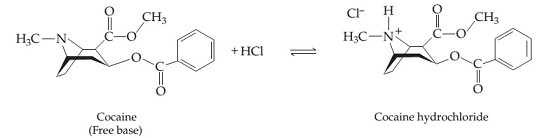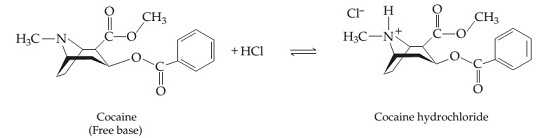# Problem: The "free-base" form of cocaine (C17H21NO4) and its protonated hydrochloride form (C17H22ClNO4) are shown below; the free-base form can be converted to the hydrochloride form with one equivalent of HCl. For clarity, not all the carbon and hydrogen atoms are shown; each vertex represents a carbon atom with the appropriate number of hydrogen atoms so that each carbon makes four bonds to other atoms.How many mL of a concentrated 18.6 MHCl aqueous solution would it take to convert 1.00 kilograms (a "kilo") of the free-base form of cocaine into its hydrochloride form?

###### FREE Expert Solution

We’re being asked to determine the volume (in mL) of 18.6 M HCl aqueous solution would it take to convert 1.00 kg of cocaine into its hydrochloride form.

We're given the following reaction:\

Recall Molarity (M):

We will calculate the volume of HCl in the following sequence of calculation:

96% (53 ratings)###### Problem Details

The "free-base" form of cocaine (C17H21NO4) and its protonated hydrochloride form (C17H22ClNO4) are shown below; the free-base form can be converted to the hydrochloride form with one equivalent of HCl. For clarity, not all the carbon and hydrogen atoms are shown; each vertex represents a carbon atom with the appropriate number of hydrogen atoms so that each carbon makes four bonds to other atoms.How many mL of a concentrated 18.6 MHCl aqueous solution would it take to convert 1.00 kilograms (a "kilo") of the free-base form of cocaine into its hydrochloride form?